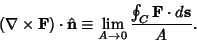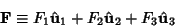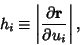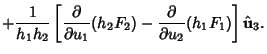## Curl

The curl of a Tensor field is given by(1)

whereis the Levi-Civita Tensor and ;'' is the Covariant Derivative. For a Vector Field, the curl is denoted(2)

andis normal to the Plane in which the circulation'' is Maximum. Its magnitude is the limiting value of circulation per unit Area,(3)

Let(4)

and(5)

then(6)

Special cases of the curl formulas above can be given for Curvilinear Coordinates.

Arfken, G. Curl,.'' §1.8 in Mathematical Methods for Physicists, 3rd ed. Orlando, FL: Academic Press, pp. 42-47, 1985.